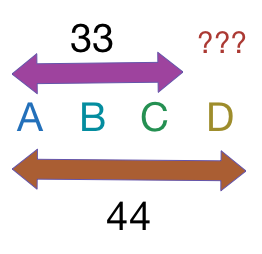# 4 Numbers AverageI am thinking of $4$ numbers.
The average of the $4$ numbers is $44.$
The average of the first $3$ numbers is $33.$

What is the value of the $4^\text{th}$ number?

×